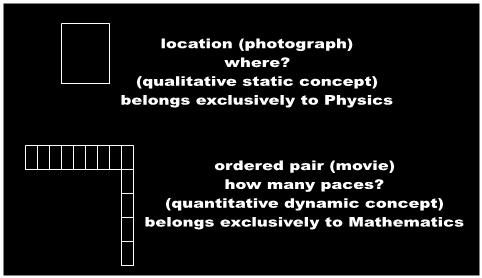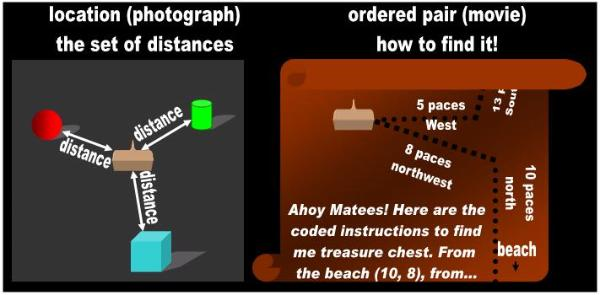One of the popular definitions of the word point is the ordered pair:

There are four main definitions of a point. They are the dot, the exact location, the
ordered pair, and the node.”  

Coordinate Geometry: Points as Ordered Pairs… Each point in the plane is now a
location in the Cartesian plane and is represented by an ordered pair.”  

I treat the ordered-pair point separately from the location point because a location is not the same thing as
a pair of numbers that supposedly represent a location. In fact, we will find out that they have nothing in
common.

In general, the mathematicians define an ordered pair as:

a collection of two objects such that one can be distinguished as the first element
and the other as the second element. An ordered pair with first element a and second
element b is usually written as (a, b).”  

In particular, the ordered pair is a set of two numbers that specifies the location of a point on a plane:

“ an ordered pair is a pair of numbers in parentheses used to locate an exact location
on a coordinate plane. The first number, represented by the variable x, tells you how
far along the x-axis the point is(to the right or left, depending on the 'polarity'/direction
of the variable). The second number does the same, just along the y-axis (up and
down).” 

“ On the coordinate plane, the pair of numbers giving the location of a point (ordered
pair).”  

In other words, under the ordered pair proposal, the mathematical physicists are once again attempting to
pass a point for a location. The symbol (x, y) depicts two ‘coordinates,’ where x typically stands for latitude
and y for longitude. Think of locating a geographical point such as the City of Paris on a map. The
‘coordinates’ for Paris are roughly: latitude 48º and longitude 2º. Therefore, the mathematicians would
represent this specific geographical point with the ordered pair (48, 2).

The first problem is that an ordered pair is NOT equivalent to a geometric figure known as a point. In fact,
the ordered pair is not equivalent to the concept location either. The word location does not even belong
in Mathematics because it is a static, qualitative concept: the set of gaps that separates one object from
the remaining ones in the Universe. Mathematics deals exclusively with dynamic, quantitative concepts.
Mathematics has no use whatsoever for static objects such as dots and cubes or for static concepts such
as  flat,  straight, and  direction. Mathematics has no authority to tell us anything about the physical
universe because it is restricted to counting, accounting, and magnitudes. Perhaps in religion and in
ordinary speech.the words point and location are defined by two numbers, but not in Science.

If location is a still image, an ordered pair is a motion picture (Fig. 1). An ordered pair is conceptually
comprised of many locations. An ordered pair does not tell us WHAT a treasure chest IS. It tells us how
many paces the pirate must walk, first in one direction and then in another, if he wants to find a treasure
chest (Fig. 2).

More perplexing and confusing, the ordered-pair point of Mathematics invokes two number lines. If as
the mathematicians allege, we need two coordinates – latitude and longitude – to specify or define a
point, how is a point different from a plane? In the Symposium of Mathematics going on next door, the
mathematicians claim that they need two lines to define a plane:

“A plane is determined by two intersecting lines.”  

“a plane is uniquely determined by…two different lines which intersect”  

This is the justification the mathematicians give for why a plane is 2-D. Wasn't the point zero or non-
dimensional? How did this 0-D, ‘no part’ point suddenly acquire the two dimensions of the ordered pair
(x, y)?

In fact, just to show how inconsistent mathematicians are, here’s an example of how the idiots of
Mathematics chase their tails around. According to one site, we need zero coordinates or numbers to
locate a point:

“ A line is one-dimensional, since one number, the distance from zero, tells you where
you are. A plane is two-dimensional, since you need x and y to locate a point. A point
is dimensionless. It consists only of location, so it’s only possible to be one place if
you’re on a point – you don’t need any extra numbers to tell you where you are. ”  

[Appalling logic! But this is what these people are taught by rote at the university! This
is what they later repeat without ever having analyzed it!]

So do we need two numbers to specify a point or none? This shows you what a bunch of morons the
mathematicians are! The 0-D versus 2-D dichotomy of Mathematics is solely an issue of language and
has nothing to do with equations or numbers. The mathematicians run around in circles because
Mathematics is a discipline that either refuses to define words or uses them consistently.

…And thank the Almighty that the point decided not to sit on a box, for otherwise two variables would
be insufficient to establish its location. We would now have to define the point as an ordered triplet:

“ Sometimes people want to describe a point in three dimensions...To do this, they
need to use a triplet of numbers like  (1, 2, -5)”  

“ Intuitively one can understand a location in the Cartesian 3D space. This location
could be described with three real number coordinates: for instance P = (2, 6, 9).”   

Imagine if the hapless ET has to wait until the stupid relativist finds a third stick in the woods to
understand what a point is.

Again, the mathematicians are confusing what a point is (i.e., a dot) with the static concept location.
Then they confuse the location with the coded instructions necessary to arrive at a location from a
reference point. These three notions are mutually exclusive, yet the idiots of Mathematics use them
interchangeably throughout the dissertation. If the mathematician claims on the one hand that all
geometric figures and objects are made of points, then he surely can’t use the ordered pair as a building
block. You can make a far-fetched argument that an elephant is made of points. It is absolute lunacy to
propose that an elephant is comprised of ordered pairs or locations. It is the inconsistent use of the
definition of point during a given presentation that prevents the mathematicians from ‘defining’ what a
point is. If he defines it as a dot, he should not be allowed to use it as ‘the location of the dot’. Certainly,
the mathematician can't fit an ‘infinite’ number of dots on a line segment. If he defines it as a location, he
can’t use it as ‘the number of steps needed to get to a location’ (i.e., ordered pair). If he defines it as an
ordered pair or as a location, he can’t use it to build geometric figures. So, he prefers to tell you that the
point is best left undefined for otherwise it would ruin his religion.

Therefore, two intersecting coordinates or two numbers (ordered pair) define neither a point nor a
location, more so if the lines are ultimately neither coordinates nor dimensions, but number lines.
Nevertheless, an elephant is not comprised either of locations or ordered pairs. An elephant is
comprised of atoms, but even this fact is immaterial to my next argument. When we talk of location,
we are implicitly putting something there. We cannot conceptualize location by itself without an object.
If there is a location on an elephant it is because a piece of skin occupies that volume of space.
However, when we point to the object elephant, we are treating this object as if it consisted of a single
piece. Only the concept elephant can at best be subjected to the idiotic mereologic (i.e., parts of a whole)
analysis of Philosophy. In science, an object, any object, is regarded as a single piece. Only the idiots of
Philosophy spend eons talking about temporal parts and parts of a whole.
 Fig. 1   Location ≠ Ordered Pair

 Kill Bill
doing justice with the right coordinates
 A location is a qualitative concept that belongs exclusively to Physics. Mathematicians have no use for location. Conversely, an ordered pair consists of a pair of number lines. An ordered pair is a dynamic, quantitative concept. It belongs exclusively to Mathematics.
 Fig. 2Location ≠ Ordered PairShoot guys!I betcha you're such lousy shots that you can't hit anywhere near the apple.Ha, ha, ha!
 Theordered-pairpoint
 Adapted for the Internet from:Why God Doesn't Exist________________________________________________________________________________________